# RD Sharma Solutions for Class 6 Maths Chapter 7: Decimals Objective Type Questions

The main concept that has greater importance in this Chapter is Decimals. The students need to understand the concepts which help them to score good marks in the higher classes as well. RD Sharma Solutions for Class 6 has answers for the objective type questions which are given at the end of this Chapter. Using RD Sharma Solutions for Class 6 Maths Chapter 7 Decimals Objective Type Questions PDF which are provided here, students can self analyse the areas of weaknesses which are important from the exam point of view.

## RD Sharma Solutions for Class 6 Maths Chapter 7: Decimals Objective Type Questions Download PDF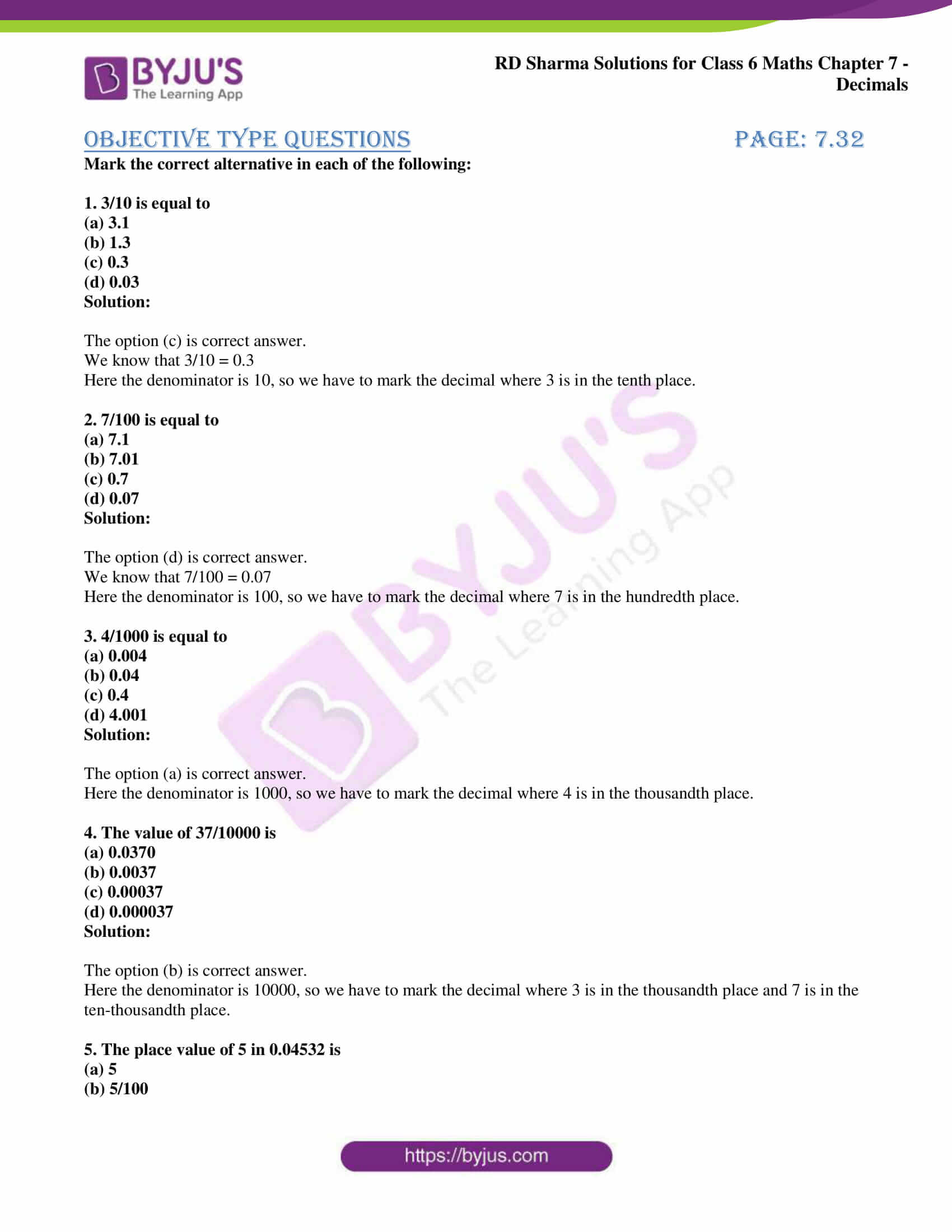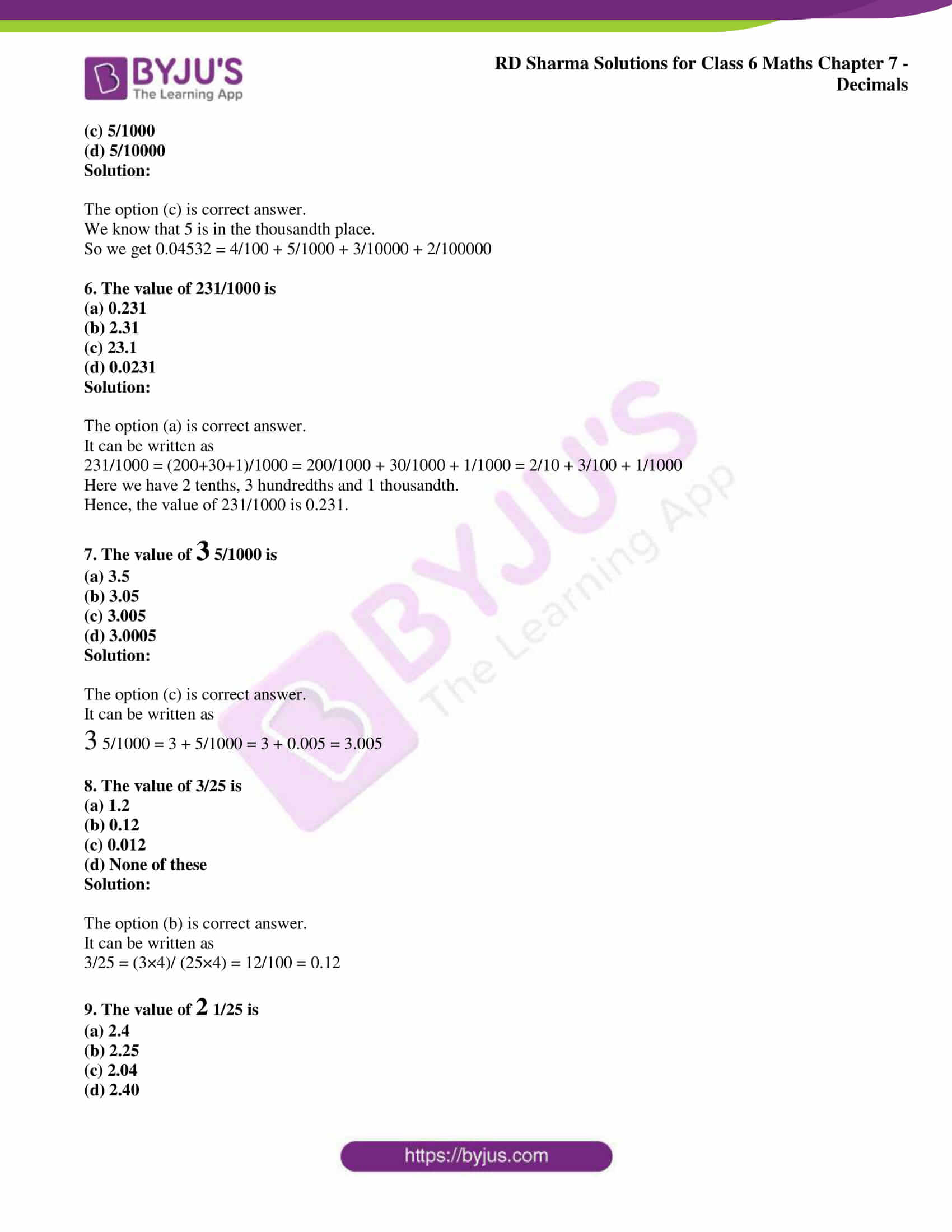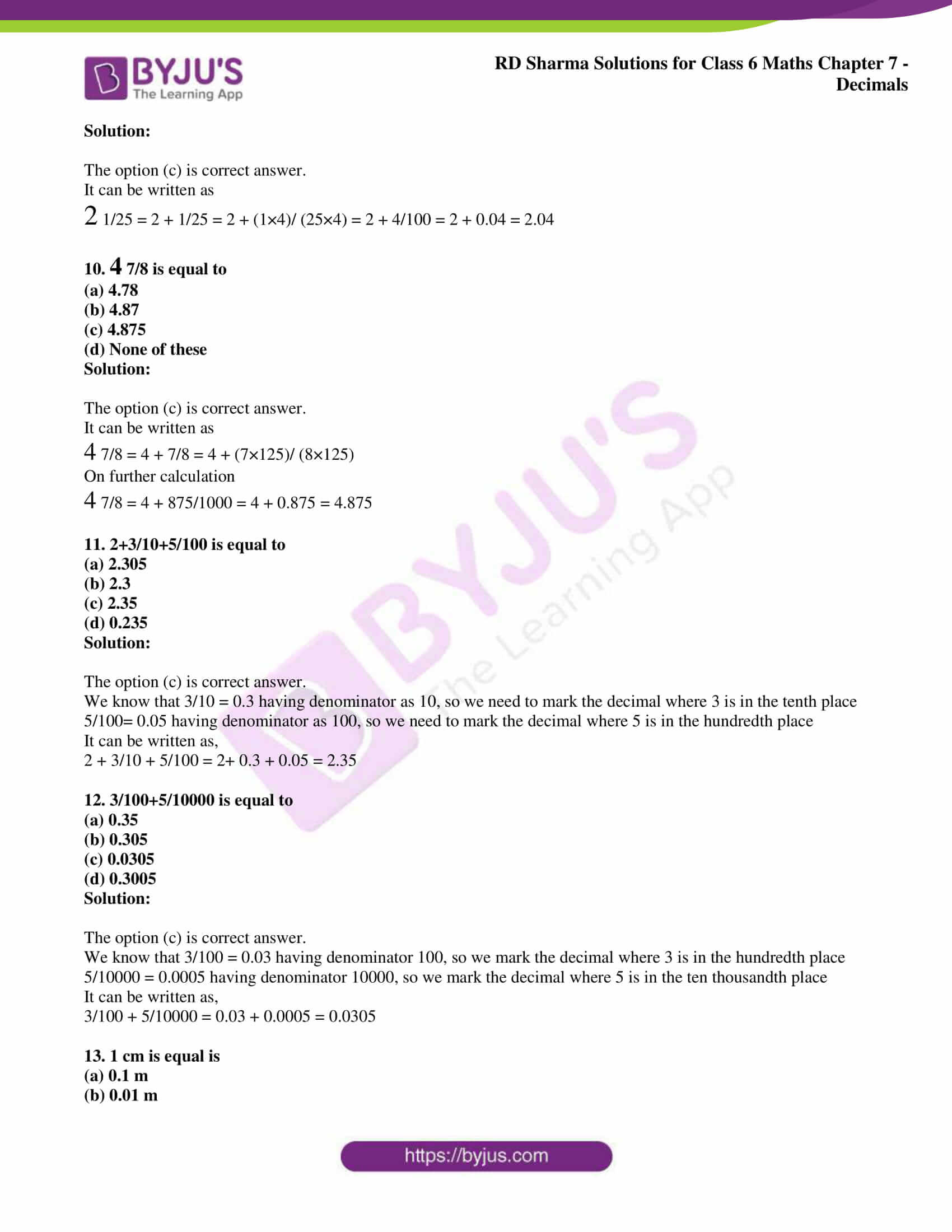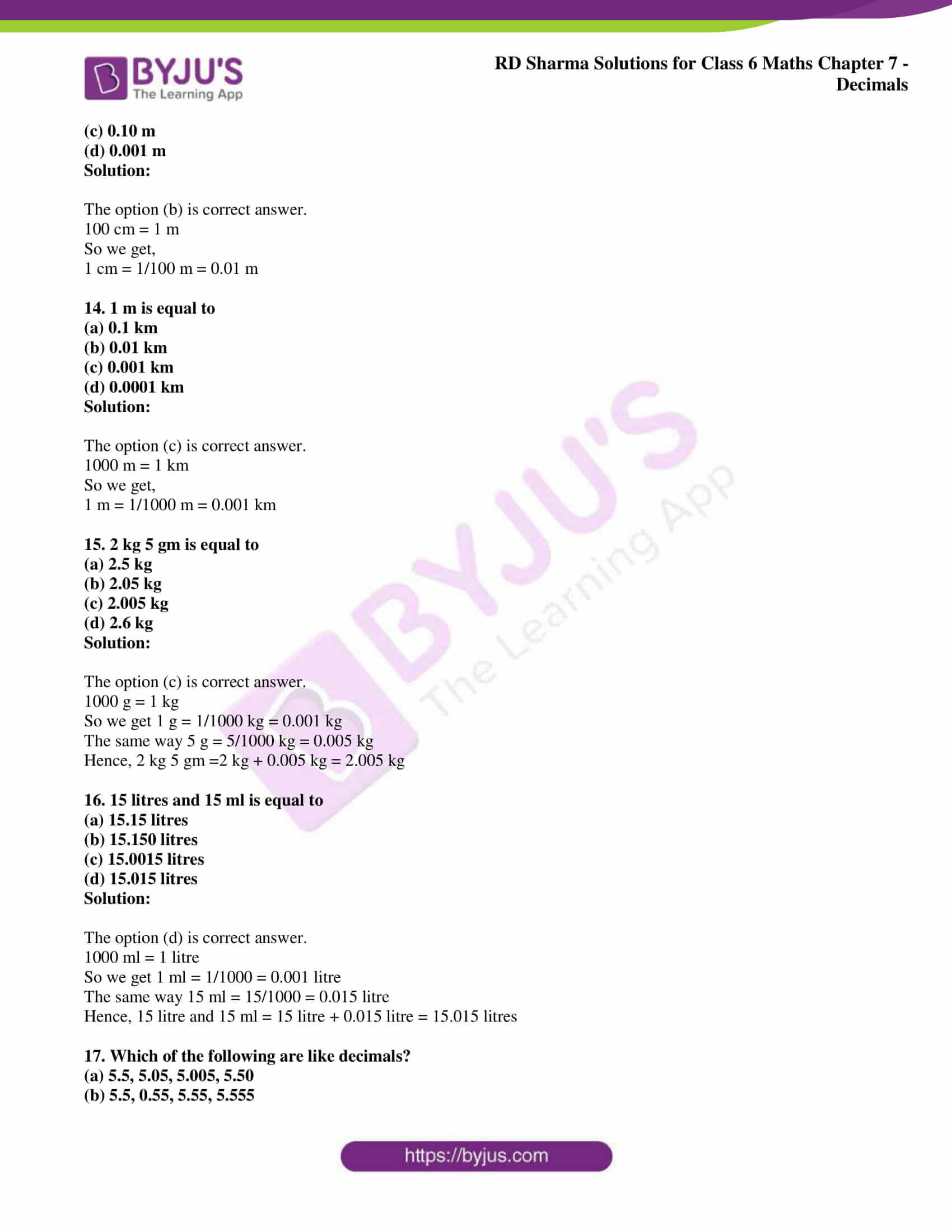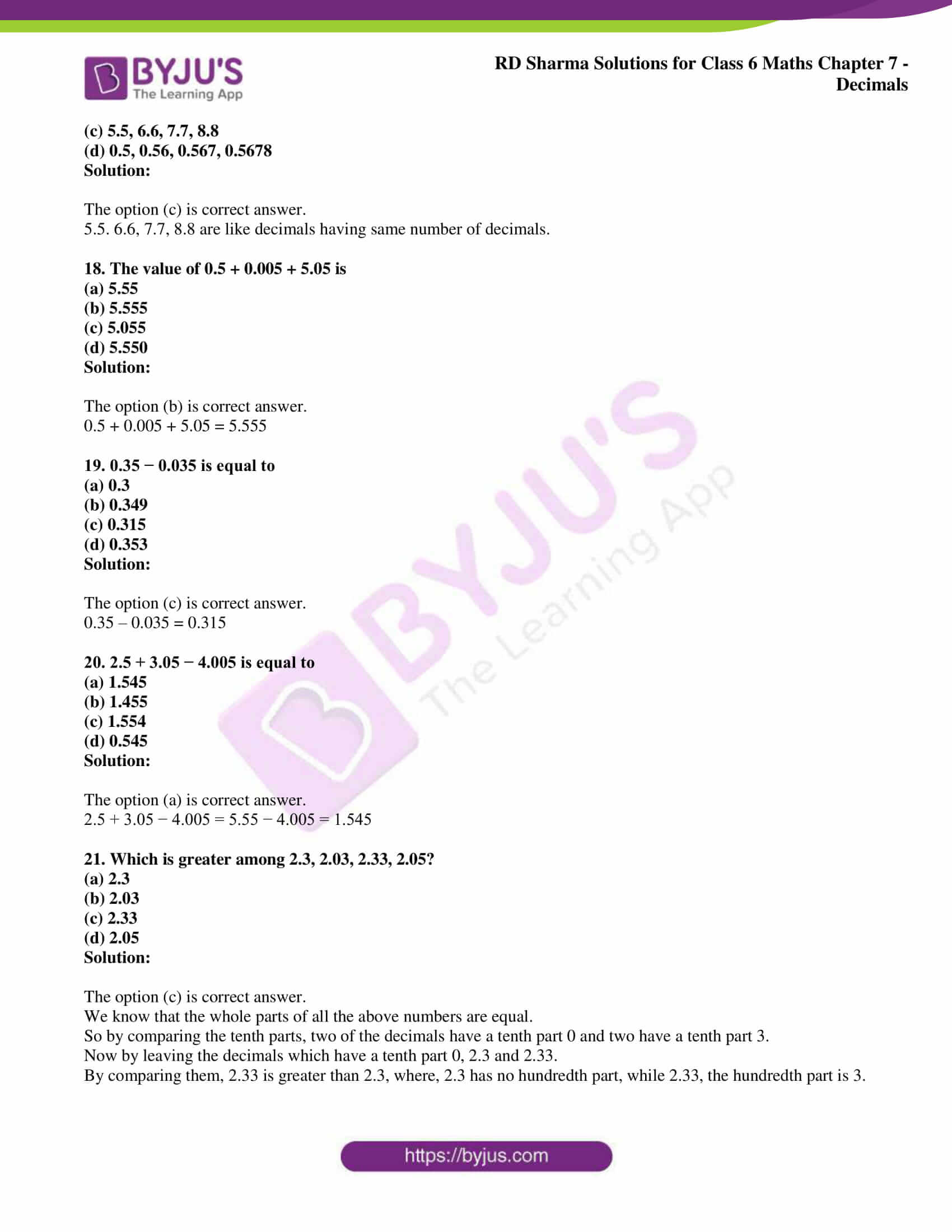### Access answers to Maths RD Sharma Solutions for Class 6 Chapter 7: Decimals Objective Type Questions

Mark the correct alternative in each of the following:

1. 3/10 is equal to
(a) 3.1
(b) 1.3
(c) 0.3
(d) 0.03

Solution:

The option (c) is correct answer.

We know that 3/10 = 0.3

Here the denominator is 10, so we have to mark the decimal where 3 is in the tenth place.

2. 7/100 is equal to
(a) 7.1
(b) 7.01
(c) 0.7
(d) 0.07

Solution:

The option (d) is correct answer.

We know that 7/100 = 0.07

Here the denominator is 100, so we have to mark the decimal where 7 is in the hundredth place.

3. 4/1000 is equal to
(a) 0.004
(b) 0.04
(c) 0.4
(d) 4.001

Solution:

The option (a) is correct answer.

Here the denominator is 1000, so we have to mark the decimal where 4 is in the thousandth place.

4. The value of 37/10000 is
(a) 0.0370
(b) 0.0037
(c) 0.00037
(d) 0.000037

Solution:

The option (b) is correct answer.

Here the denominator is 10000, so we have to mark the decimal where 3 is in the thousandth place and 7 is in the ten-thousandth place.

5. The place value of 5 in 0.04532 is
(a) 5
(b) 5/100
(c) 5/1000
(d) 5/10000

Solution:

The option (c) is correct answer.

We know that 5 is in the thousandth place.

So we get 0.04532 = 4/100 + 5/1000 + 3/10000 + 2/100000

6. The value of 231/1000 is
(a) 0.231
(b) 2.31
(c) 23.1
(d) 0.0231

Solution:

The option (a) is correct answer.

It can be written as

231/1000 = (200+30+1)/1000 = 200/1000 + 30/1000 + 1/1000 = 2/10 + 3/100 + 1/1000

Here we have 2 tenths, 3 hundredths and 1 thousandth.

Hence, the value of 231/1000 is 0.231.

7. The value of 3 5/1000 is
(a) 3.5
(b) 3.05
(c) 3.005
(d) 3.0005

Solution:

The option (c) is correct answer.

It can be written as

3 5/1000 = 3 + 5/1000 = 3 + 0.005 = 3.005

8. The value of 3/25 is
(a) 1.2
(b) 0.12
(c) 0.012
(d) None of these

Solution:

The option (b) is correct answer.

It can be written as

3/25 = (3×4)/ (25×4) = 12/100 = 0.12

9. The value of 2 1/25 is
(a) 2.4
(b) 2.25
(c) 2.04
(d) 2.40

Solution:

The option (c) is correct answer.

It can be written as

2 1/25 = 2 + 1/25 = 2 + (1×4)/ (25×4) = 2 + 4/100 = 2 + 0.04 = 2.04

10. 4 7/8 is equal to
(a) 4.78
(b) 4.87
(c) 4.875
(d) None of these

Solution:

The option (c) is correct answer.

It can be written as

4 7/8 = 4 + 7/8 = 4 + (7×125)/ (8×125)

On further calculation

4 7/8 = 4 + 875/1000 = 4 + 0.875 = 4.875

11. 2+3/10+5/100 is equal to
(a) 2.305
(b) 2.3
(c) 2.35
(d) 0.235

Solution:

The option (c) is correct answer.

We know that 3/10 = 0.3 having denominator as 10, so we need to mark the decimal where 3 is in the tenth place

5/100= 0.05 having denominator as 100, so we need to mark the decimal where 5 is in the hundredth place

It can be written as,

2 + 3/10 + 5/100 = 2+ 0.3 + 0.05 = 2.35

12. 3/100+5/10000 is equal to
(a) 0.35
(b) 0.305
(c) 0.0305
(d) 0.3005

Solution:

The option (c) is correct answer.

We know that 3/100 = 0.03 having denominator 100, so we mark the decimal where 3 is in the hundredth place

5/10000 = 0.0005 having denominator 10000, so we mark the decimal where 5 is in the ten thousandth place

It can be written as,

3/100 + 5/10000 = 0.03 + 0.0005 = 0.0305

13. 1 cm is equal is
(a) 0.1 m
(b) 0.01 m
(c) 0.10 m
(d) 0.001 m

Solution:

The option (b) is correct answer.

100 cm = 1 m

So we get,

1 cm = 1/100 m = 0.01 m

14. 1 m is equal to
(a) 0.1 km
(b) 0.01 km
(c) 0.001 km
(d) 0.0001 km

Solution:

The option (c) is correct answer.

1000 m = 1 km

So we get,

1 m = 1/1000 m = 0.001 km

15. 2 kg 5 gm is equal to
(a) 2.5 kg
(b) 2.05 kg
(c) 2.005 kg
(d) 2.6 kg

Solution:

The option (c) is correct answer.

1000 g = 1 kg

So we get 1 g = 1/1000 kg = 0.001 kg

The same way 5 g = 5/1000 kg = 0.005 kg

Hence, 2 kg 5 gm =2 kg + 0.005 kg = 2.005 kg

16. 15 litres and 15 ml is equal to
(a) 15.15 litres
(b) 15.150 litres
(c) 15.0015 litres
(d) 15.015 litres

Solution:

The option (d) is correct answer.

1000 ml = 1 litre

So we get 1 ml = 1/1000 = 0.001 litre

The same way 15 ml = 15/1000 = 0.015 litre

Hence, 15 litre and 15 ml = 15 litre + 0.015 litre = 15.015 litres

17. Which of the following are like decimals?
(a) 5.5, 5.05, 5.005, 5.50
(b) 5.5, 0.55, 5.55, 5.555
(c) 5.5, 6.6, 7.7, 8.8
(d) 0.5, 0.56, 0.567, 0.5678

Solution:

The option (c) is correct answer.

5.5. 6.6, 7.7, 8.8 are like decimals having same number of decimals.

18. The value of 0.5 + 0.005 + 5.05 is
(a) 5.55
(b) 5.555
(c) 5.055
(d) 5.550

Solution:

The option (b) is correct answer.

0.5 + 0.005 + 5.05 = 5.555

19. 0.35 − 0.035 is equal to
(a) 0.3
(b) 0.349
(c) 0.315
(d) 0.353

Solution:

The option (c) is correct answer.

0.35 – 0.035 = 0.315

20. 2.5 + 3.05 − 4.005 is equal to
(a) 1.545
(b) 1.455
(c) 1.554
(d) 0.545

Solution:

The option (a) is correct answer.

2.5 + 3.05 − 4.005 = 5.55 − 4.005 = 1.545

21. Which is greater among 2.3, 2.03, 2.33, 2.05?
(a) 2.3
(b) 2.03
(c) 2.33
(d) 2.05

Solution:

The option (c) is correct answer.

We know that the whole parts of all the above numbers are equal.

So by comparing the tenth parts, two of the decimals have a tenth part 0 and two have a tenth part 3.

Now by leaving the decimals which have a tenth part 0, 2.3 and 2.33.

By comparing them, 2.33 is greater than 2.3, where, 2.3 has no hundredth part, while 2.33, the hundredth part is 3.